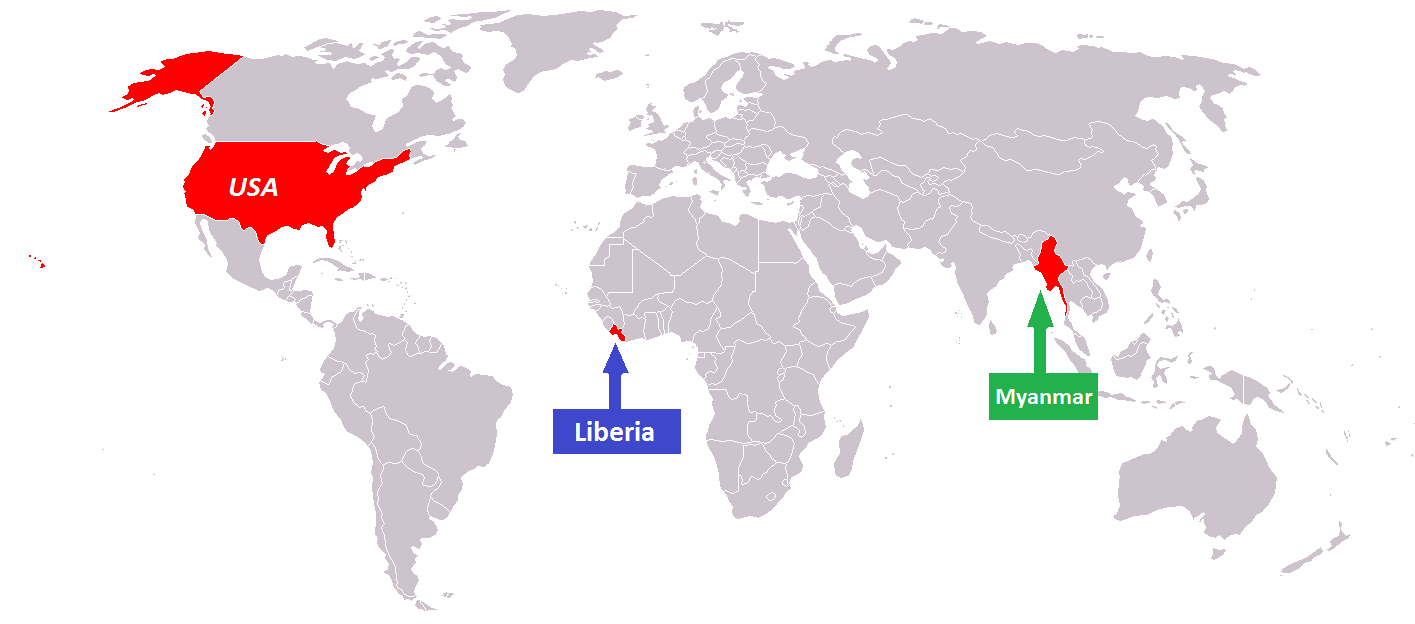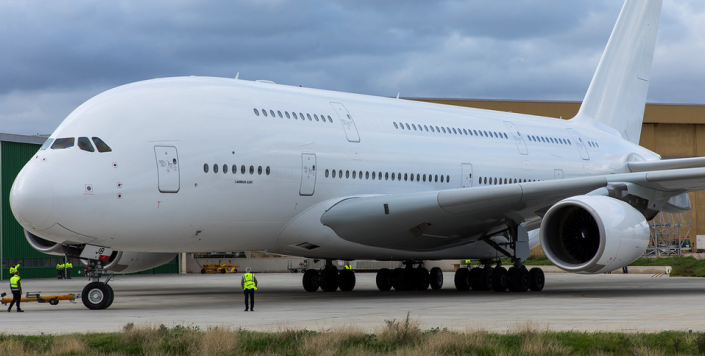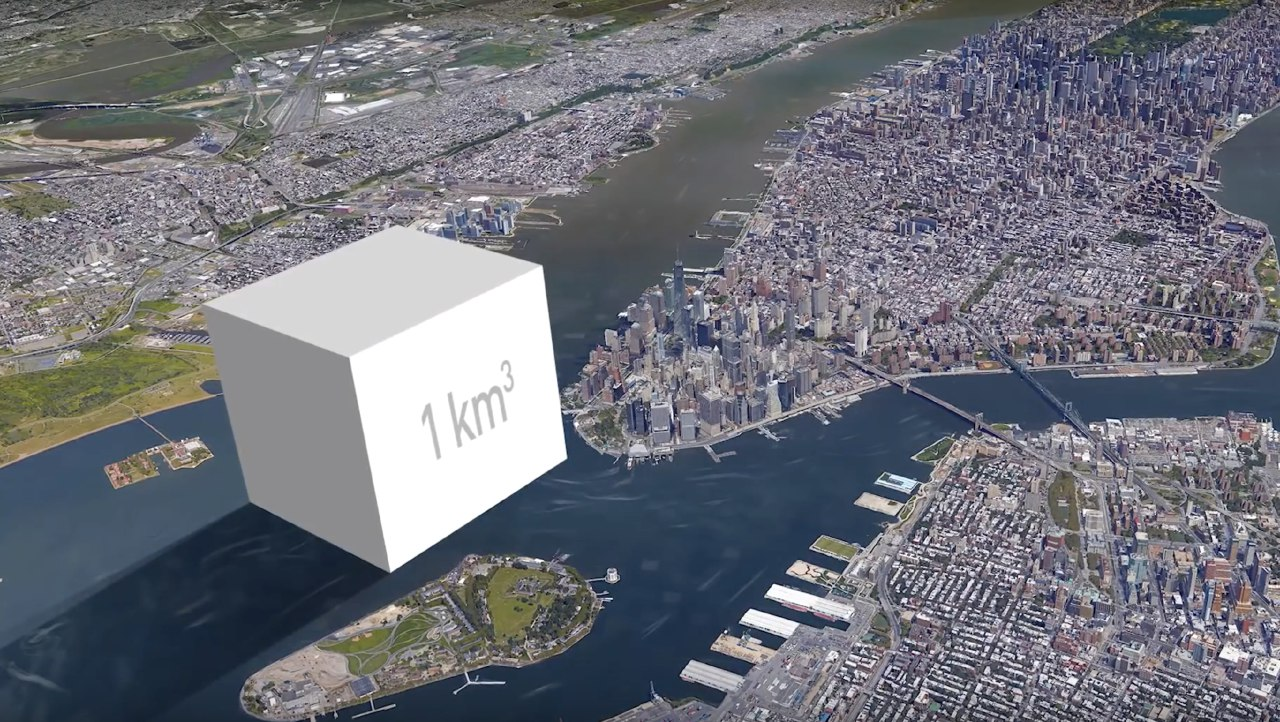A cube, a quintillion dollars coin and the power of decimalsLast week stood out by some popular discussions about minting a particularly huge bullion coin to avoid a Debt Ceiling default of the United States of America’s Federal Government. More precisely, a one Trillion USD platinum bullion coin. While I’m not an expert on American right nor do I have any opinion about if such a project is wise or not, contrary to what some Twitter commenters suggested to my thread:

To be fair, I genuinely wondered what such a bullion coin would look like if it was indeed made of one Trillion dollars worth of platinum at the current valuation. In case you’re more interested in the former legal aspects of such a project, I encourage you to go through this more law-oriented blog post from Preston Byrne, who, as an American lawyer, is I suppose much better informed about American rights than I’ll ever be.

Therefore, in this blog post, I’m going through where this idea came from, how I made my calculation and initially done a volume conversion mistake. It’s also an occasion for me to test more in-depth this $$\LaTeX$$ javascript library 1. Now that our workplace is set, let’s follow the sheep:

This whole idea started with an old article from Wired 2013, I ended up there by googling something like “$1T platimium coin volume”, and while the article is a bit old to be relevant, my main source of annoyance was: Such a coin would weigh 42,778,918 pounds – the equivalent of nearly seven Saturn V rockets – and occupy 31,947 cubic feet. As a reminder here is the map of all the countries in the World which are not officially using the metric system:So let’s start back the calculus in a system that everyone can follow. While prices keep fluctuating, one kg of platinum is currently worth around$31500, so from here we can check the conjectural weight of one Trillion worth of platinum:

$$\frac{1 T}{31.5k} = \frac{10^{12}}{31.5 \times 10^3} \approx 31.7 \times 10^6kg$$

Or 31.7k tons of platinum or between 150 and 200 years of platinum mining depending on the year you use as a reference. I can read online that one cubic meter of platinum weighs 21.45 tons, from there we can easily determine the volume of this Trillion coin:

$$\frac{31.7 \times 10^3}{21.45} \approx 1.48 \times 10^3m^3$$

And it’s here where I’ve done a dumb mistake in the rush, I wanted to find a visual to understand what 1000 $$m^3$$ would look like and in my mind one kilometer is the addition of 1000 meters. And that’s here that the power of decimals is coming in:

 $$km$$ $$10^3 m$$ $$km^2$$ $$10^3m \times 10^3m = 10^6 m^2$$ $$km^3$$ $$10^3m \times 10^3m \times 10^3m = 10^9 m^3$$

Ultimately the one trillion dollar coin would be one-third bigger than an Airbus A380 which has a total hold volume of 1134$$m^3$$. Thus not as big as Manhattan’s giant towers, but already quite significant in size.For fun and glory, let’s reverse engineer the theoretical worth price of my initial one cubic kilometer platinum bullion coin initially represented next to Manhattan island:For this nothing easier, we just have to divide the volume of this cube by the volume of our theoric Trillion dollar platinum coin:

$$\frac{10^9 m^3}{1.48 \times 10^3 m^3} \approx 6.76 \times 10^5T$$

We can now confidently say that this cube is a 0.67 quintillion dollars bullion platinum coin!

1. Which oddly enough is the only library I’m serving from a CDN, see my Privacy policy ↩︎